# Plug-in estimators of statistical functionals

Consider a sequence of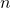independent and identically distributed random variables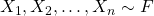. The distribution function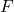is unknown but belongs to a known set of distribution functions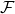. In parametric estimation,may represent a family of distributions specified by a vector of parameters, such as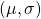in the case of the location-scale family. In nonparametric estimation,is much more broad and is subject to milder restrictions, such as the existence of moments or continuity. For example, we may defineas the family of distributions for which the mean exists or all distributions defined on the real line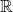.

As mentioned in my previous blog post comparing nonparametric and parametric estimation, a statistical functional is any real-valued function of the cumulative distribution function, denoted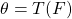. Statistical functionals can be thought of as characteristics of, and include moments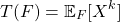and quantiles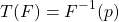as examples.

An infinite population may be considered as completely determined by its distribution function, and any numerical characteristic of an infinite population with distribution functionthat is used in statistics is a [statistical] functional of.

Wassily Hoeffding. “A Class of Statistics with Asymptotically Normal Distribution.” Ann. Math. Statist. 19 (3) 293 – 325, September, 1948.

This blog post aims to provide insight into estimators of statistical functionals based on a sample ofindependent and identically random variables, known as plug-in estimators or empirical functionals.

## Expectation with respect to CDF

Many statistical functionals are expressed as the expectation of a real-valued function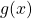with respect to. That is, for a single random variable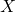,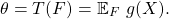For example, the population mean can be expressed as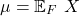and the population variance can be expressed as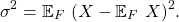What does it mean to take the expectation of a function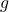of a random variablewith respect to a distribution function? Formally,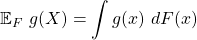which takes the form of a Riemann-Stieltjes integral. We can re-express this integral so that it takes a more familiar form.

1. Ifis discrete,has a corresponding mass function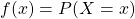such that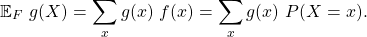2. Ifis continuous,has a corresponding density function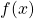such that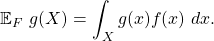This is just the usual form of the expectation of a function of a random variable per the Law of the unconcious statistician. The extension to two or more independent random variables is straight-forward,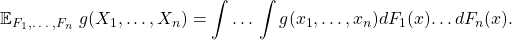## Empirical cumulative distribution function

A natural estimator ofis the empirical cumulative distribution function (ECDF), defined as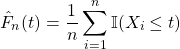where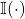is an indicator function taking the value 1 if its argument is true and 0 otherwise. That is, the estimated probability that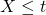is the sample proportion of observations less than or equal to. Then, for a given value of, it is easy to show that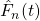is a consistent estimator of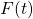.

Let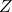represent the number of‘s less than or equal to. Then,is distributed according to a Binomial distribution withtrials and success probability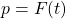. That is,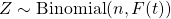. The sample estimate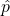of the success probability is then. The central limit theorem tells us that for a sample proportion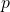,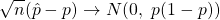and thus, it follows that for fixed,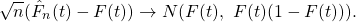Note that a stronger result is available for allsimultaneously,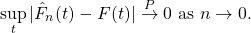The ECDF can be implemented in R from scratch using the following code.

set.seed(12345)

library(tidyverse)

# Generate n = 100 observations from N(5, 1)
n = 100
X = rnorm(n, mean = 5, sd = 1)

# Specify range of x's for ECDF
X_min = min(X) - 1
X_max = max(X) + 1

# Create a sequence of t's to evaluate ECDF
t_eval = seq(X_min, X_max, 0.01)

# Estimate ECDF from scratch
Fn <- c()
for (t in t_eval){

Ix <- ifelse(X <= t, 1, 0)    # I(Xi <= x)
Fx <- (1/n) * sum(Ix)         # Defn of Fn(x)
Fn <- append(Fn, Fx)          # Add result to Fn vector

}

# Plot ECDF
qplot(x = t_eval, y = Fn, geom = 'step') +
labs(x = 't', y = 'ECDF(t)', title = 'ECDF of random sample of size n = 100 from N(5, 1)') +
lims(x = c(2, 8)) +
theme_bw()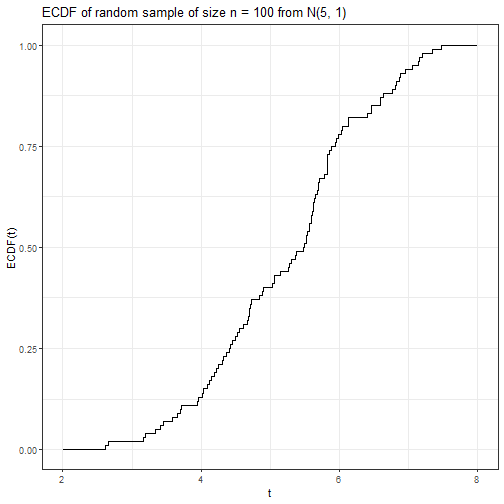Alternatively, the ECDF can be generated using R’s built-in ecdf function, which provides convenient methods such as plotting and quantiles.

Fn <- ecdf(X)
plot(Fn)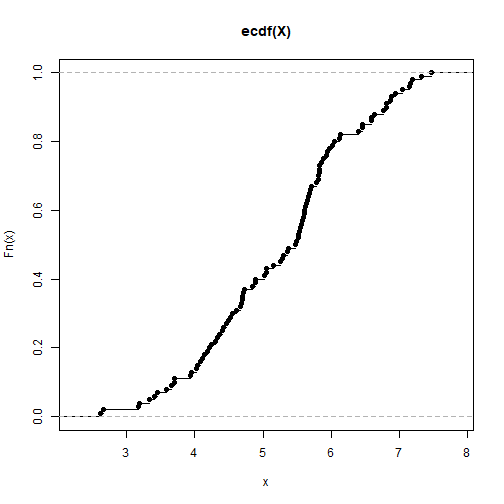quantile(Fn, 0.75)

##     75%
## 5.90039


## Empirical functionals, or plug-in estimators

Statistical functionals can be naturally estimated by an empirical functional which substitutes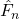forsuch that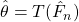. For this reason, empirical functionals are also commonly referred to as plug-in estimators.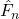is a valid, discrete CDF which assigns mass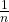to each of the observed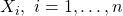. For a random variable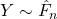,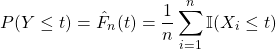and,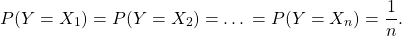Whentakes the form of an expectation with respect to, replacingwithyields,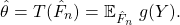Sinceis a discrete distribution,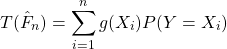suggests that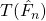is just the sample average of thetransformed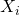,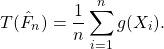As an example, the sample expectation and variance can be easily expressed as plug-in estimators: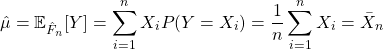mu_hat = sum(1/n * X)
mu_hat

##  5.245197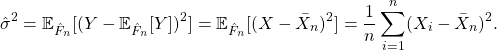sigma2_hat = sum(1/n * (X - sum(1/n * X))^2)
sigma2_hat

##  1.230199


Note that while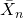is the standard, unbiased estimator of the population mean,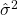is the biased estimator of the population variance featuring a denominator of.

Not all empirical functionals, or plug-in estimators, are unbiased! However, when the statistical functional takes a special form, known as an expectation functional, an unbiased estimator can always be constructed regardless of the form of.